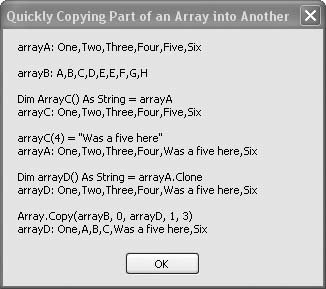# Recipe 8.8. Quickly Copying Part of an Array into Another

#### Problem

You want to copy elements of one array into another without having to move the items one at a time.

#### Solution

Sample code folder: Chapter 08\ CopyingArrays

Use the Array.Copy() method to copy a sequential subset of one array to another array of the same type. Or, if the entire array is to be copied, use the array's Clone() method. Assign one array directly to another only if you want both variables to reference the same contents in memory.

#### Discussion

This recipe explores several ways to copy elements from one array to another, and one way that appears to do a copy but doesn't. It's important to know the difference between these various techniques. The following block of code demonstrates all of them and displays the results in a message box:

` Dim result As New System.Text.StringBuilder Dim arrayA( ) As String = _    {"One", "Two", "Three", "Four", "Five", "Six"} result.Append("arrayA: ").AppendLine(Join(arrayA, ",")) Dim arrayB( ) As String = _    {"A", "B", "C", "D", "E", "E", "F", "G", "H"} result.AppendLine( ) result.Append("arrayB: ").AppendLine(Join(arrayB, ",")) ' ----- Make a reference copy. Dim arrayC( ) As String = arrayA result.AppendLine( ) result.AppendLine("Dim arrayC( ) As String = arrayA") result.Append("arrayC: ").AppendLine(Join(arrayC, ",")) arrayC(4) = "Was a five here" result.AppendLine( ) result.AppendLine("arrayC(4) = ""Was a five here""") result.Append("arrayA: ").AppendLine(Join(arrayA, ",")) ' ----- Make a full, unique copy of all elements. Dim arrayD( ) As String = arrayA.Clone result.AppendLine( ) result.AppendLine("Dim arrayD( ) As String = arrayA.Clone") result.Append("arrayD: ").AppendLine(Join(arrayD, ",")) ' ----- Copy elements by position. Array.Copy(arrayB, 0, arrayD, 1, 3) result.AppendLine( ) result.AppendLine("Array.Copy(arrayB, 0, arrayD, 1, 3)") result.Append("arrayD: ").AppendLine(Join(arrayD, ",")) MsgBox(result.ToString( )) `

Let's break down this code into smaller chunks so we can take a closer look. The first three sections create two string arrays, arrayA and arrayB, containing simple strings so we can follow the action later. The first line of the next section is where it gets interesting:

` Dim arrayC( ) As String = arrayA `

This appears to be an array copy command, but it isn't. The two array names both reference the same contents in memory. In other words, the reference to the array is copied, not the array itself. The code in the next section demonstrates this clearly:

` arrayC(4) = "Was a five here" result.AppendLine( ) result.AppendLine("arrayC(4) = ""Was a five here""") result.Append("arrayA: ").AppendLine(Join(arrayA, ",")) `

The new string is assigned to arrayC(4), but when the contents of arrayA are formatted for display the new string appears there, too. As Figure 8-8 shows, the new string appears as an element of both arrayA and arrayC.

##### Figure 8-8. Various ways to copy data between arraysThe next-to-last code section demonstrates the proper way to truly copy an entire array to another. The array's Clone() method returns a clone, or identical duplicate, of the original array. The result is that the array's contents are copied to a new place in memory. In the example code, the reference to the cloned copy of the array is assigned to arrayD:

` Dim arrayD( ) As String = arrayA.Clone result.AppendLine( ) result.AppendLine("Dim arrayD( ) As String = arrayA.Clone") result.Append("arrayD: ").AppendLine(Join(arrayD, ",")) `

Finally, the last code section demonstrates the use of the Array class's Copy() method to copy part of one array to another. In this case both arrays must exist before the copy, and the indexes must point to real locations within the arrays. There are several overloaded versions of the Copy() method. The version shown here lets you move array elements starting at a given indexed position to any position in the destination array, and the number of elements to copy limits how much data is copied:

` Array.Copy(arrayB, 0, arrayD, 1, 3) result.AppendLine( ) result.AppendLine("Array.Copy(arrayB, 0, arrayD, 1, 3)") result.Append("arrayD: ").AppendLine(  Join(arrayD, ",")) `

arrayB's contents, starting at index 0, are copied into arrayD, starting at index 1, and three items are copied. If you've followed along carefully as these sections of code manipulate the contents of the arrays, you'll see that the result shown in Figure 8-8 does verify this copy action.Visual Basic 2005 Cookbook: Solutions for VB 2005 Programmers (Cookbooks (OReilly))
ISBN: 0596101775
EAN: 2147483647
Year: 2006
Pages: 400

Similar book on Amazon